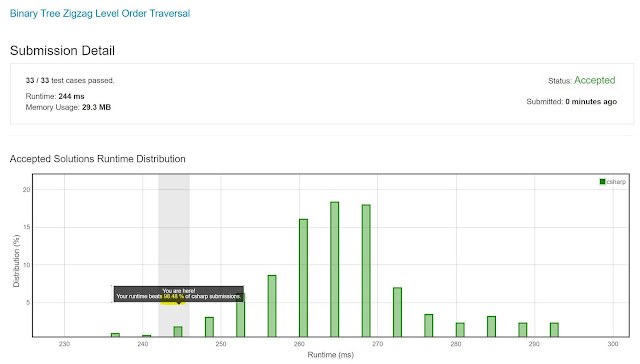## Posts

Showing posts from September, 2019

### Binary Tree Zigzag Level Order Traversalhttps://leetcode.com/problems/binary-tree-zigzag-level-order-traversal/ Given a binary tree, return the  zigzag level order  traversal of its nodes' values. (ie, from left to right, then right to left for the next level and alternate between). For example: Given binary tree  [3,9,20,null,null,15,7] , 3 / \ 9 20 / \ 15 7 return its zigzag level order traversal as: [ , [20,9], [15,7] ] Solution: Level-traversal using a Queue + DFS On enqueue put the elements on a list Apply list.reverse for the odd-levels Works fast. Code's below, cheers, ACC. public class Solution {     public IList<IList<int>> ZigzagLevelOrder(TreeNode root)     {         if (root == null) return new List<IList<int>>();         List<IList<int>> retVal = new List<IList<int>>();         Queue<QueueItem> queue = new Queue<QueueItem>();         QueueItem qi = new QueueItem(root,

### HackerRank: Prime DatesFun problem from HackerRank, and this time the task is to FIX the BUGS in a code that someone else wrote - boy that really hits close to home! https://www.hackerrank.com/challenges/prime-date/problem The code that needs to be fixed is down below (the original code). You can only modify no more than 5 lines, so here are some hints: a) The leap year calculation is wrong b) The conversion from date to an integer is wrong c) The logic to increment the date (right after the call to the LeapYear function) is wrong Give it a try, for me it worked out! Cheers, ACC. PS: that's in C++... I'm usually a C# programmer. #include <bits/stdc++.h> using namespace std; int month; void updateLeapYear(int year) { if(year % 400 == 0) { month = 28; } else if(year % 100 == 0) { month = 29; } else if(year % 4 == 0) { month = 29; } else { month = 28; } } void storeMonth() { month = 31; month = 2

### Maximum Number of Balloons#431 in my solved list:  https://leetcode.com/problems/maximum-number-of-balloons/ Given a string  text , you want to use the characters of  text  to form as many instances of the word  "balloon"  as possible. You can use each character in  text   at most once . Return the maximum number of instances that can be formed.   Example 1: Input: text = "nlaebolko" Output: 1 Example 2: Input: text = "loonbalxballpoon" Output: 2 Example 3: Input: text = "leetcode" Output: 0 Constraints: 1 <= text.length <= 10^4 text  consists of lower case English letters only. Solution is as follows:  - Count the number of occurrences of the "balloon" letters in the input string  - For "l" and "o", divide the count by 2  - If the balloon string is not fully covered, return 0  - Return the min number across all occurrences Code is below, cheers, ACC. public class Solution {     public int M

### Day Of The Week

This is an one-liner. Problem:  https://leetcode.com/problems/day-of-the-week/ Given a date, return the corresponding day of the week for that date. The input is given as three integers representing the  day ,  month  and  year  respectively. Return the answer as one of the following values  {"Sunday", "Monday", "Tuesday", "Wednesday", "Thursday", "Friday", "Saturday"} . Example 1: Input: day = 31, month = 8, year = 2019 Output: "Saturday" Example 2: Input: day = 18, month = 7, year = 1999 Output: "Sunday" Example 3: Input: day = 15, month = 8, year = 1993 Output: "Sunday" Constraints: The given dates are valid dates between the years  1971  and  2100 . Here is the one-liner:             return (new DateTime(year, month, day)).DayOfWeek.ToString(); Cheers, Boris